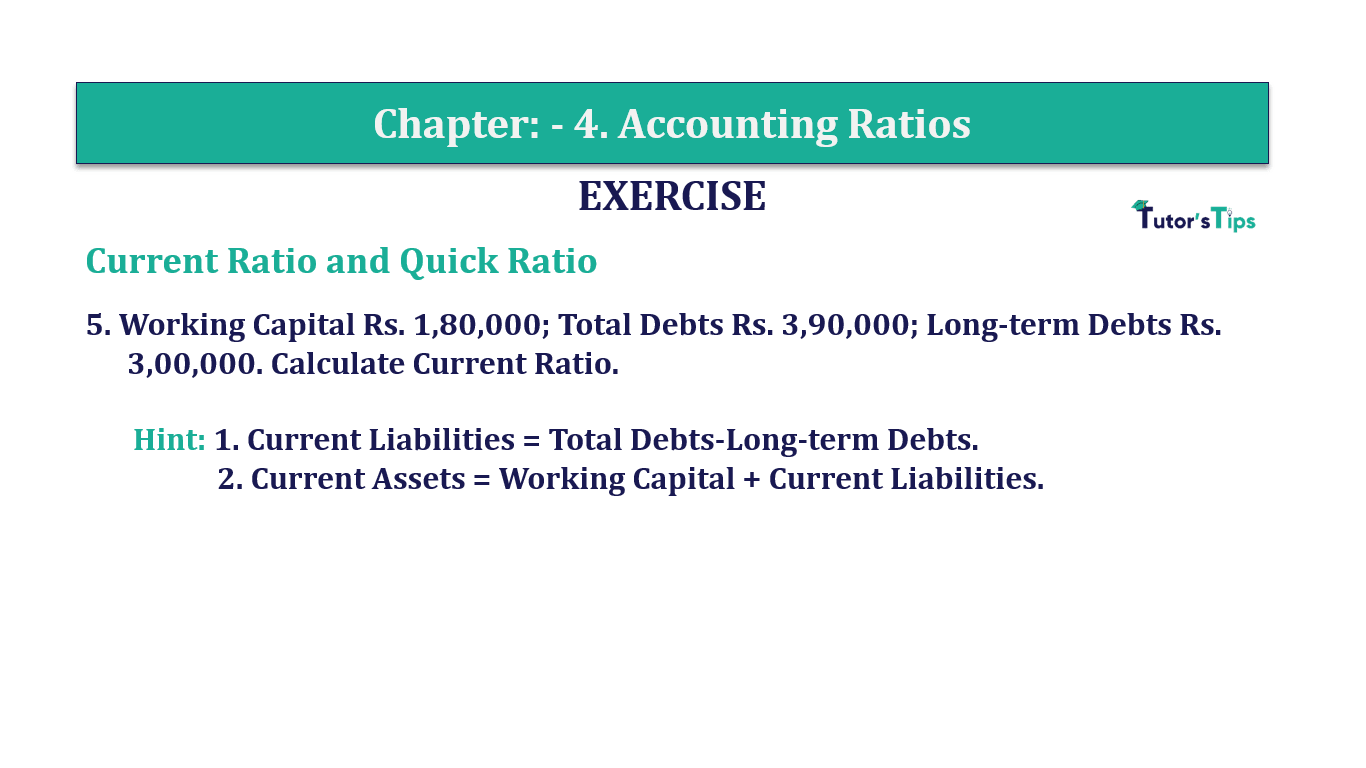# Question 5 Chapter 4 of +2-B – T.S. Grewal 12 ClassQuestion No. 5 - Chapter No.4 - T.S. Grewal +2 Book Part B

Question 5 Chapter 4 of +2-B

Current Ratio and Quick Ratio

5. Working Capital Rs. 1,80,000; Total Debts Rs. 3,90,000; Long-term Debts Rs.
3,00,000. Calculate Current Ratio.

Hint: 1. Current Liabilities = Total Debts-Long-term Debts.
2. Current Assets = Working Capital + Current Liabilities.

### The solution of Question 5 Chapter 4 of +2-B: –

 Total Debts =Rs. 3,90,000
 Long-term Debts = Rs. 3,00,000
 Current Liabilities= Total Debts – Long-term Debts
 Rs. 3,90,000 – Rs. 3,00,000
 Rs.90,000

 Working Capital =Current Assets – Current Liabilities
 Rs. 1,80,000= Current Assets – Rs. 90,000
 Current Assets Rs. 1,80,000 – Rs. 90,000
 Rs.2,70,000

 Current Ratio= Current Assets Current Liabilities
 Current Ratio= 2,70,000 90,000

Current Ratio=4:1

Balance Sheet: Meaning, Format & Examples

Comment if you have any question.

Also, Check out the solved question of previous Chapters: –